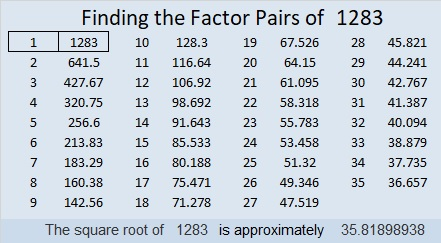# 1283 and Level 3

What is the greatest common factor of 28 and 63? If you know, then you can probably figure out this puzzle!Print the puzzles or type the solution in this excel file: 10-factors-1281-1288

Here are some facts about the number 1283:

• 1283 is a prime number.
• Prime factorization: 1283 is prime.
• The exponent of prime number 1283 is 1. Adding 1 to that exponent we get (1 + 1) = 2. Therefore 1283 has exactly 2 factors.
• Factors of 1283: 1, 1283
• Factor pairs: 1283 = 1 × 1283
• 1283 has no square factors that allow its square root to be simplified. √1283 ≈ 35.81899

How do we know that 1283 is a prime number? If 1283 were not a prime number, then it would be divisible by at least one prime number less than or equal to √1283 ≈ 35.8. Since 1283 cannot be divided evenly by 2, 3, 5, 7, 11, 13, 17, 19, 23, 29 or 31, we know that 1283 is a prime number.1283 is the sum of the seventeen prime numbers from 41 to 109,
AND it is the sum of the thirteen primes from 71 to 131.